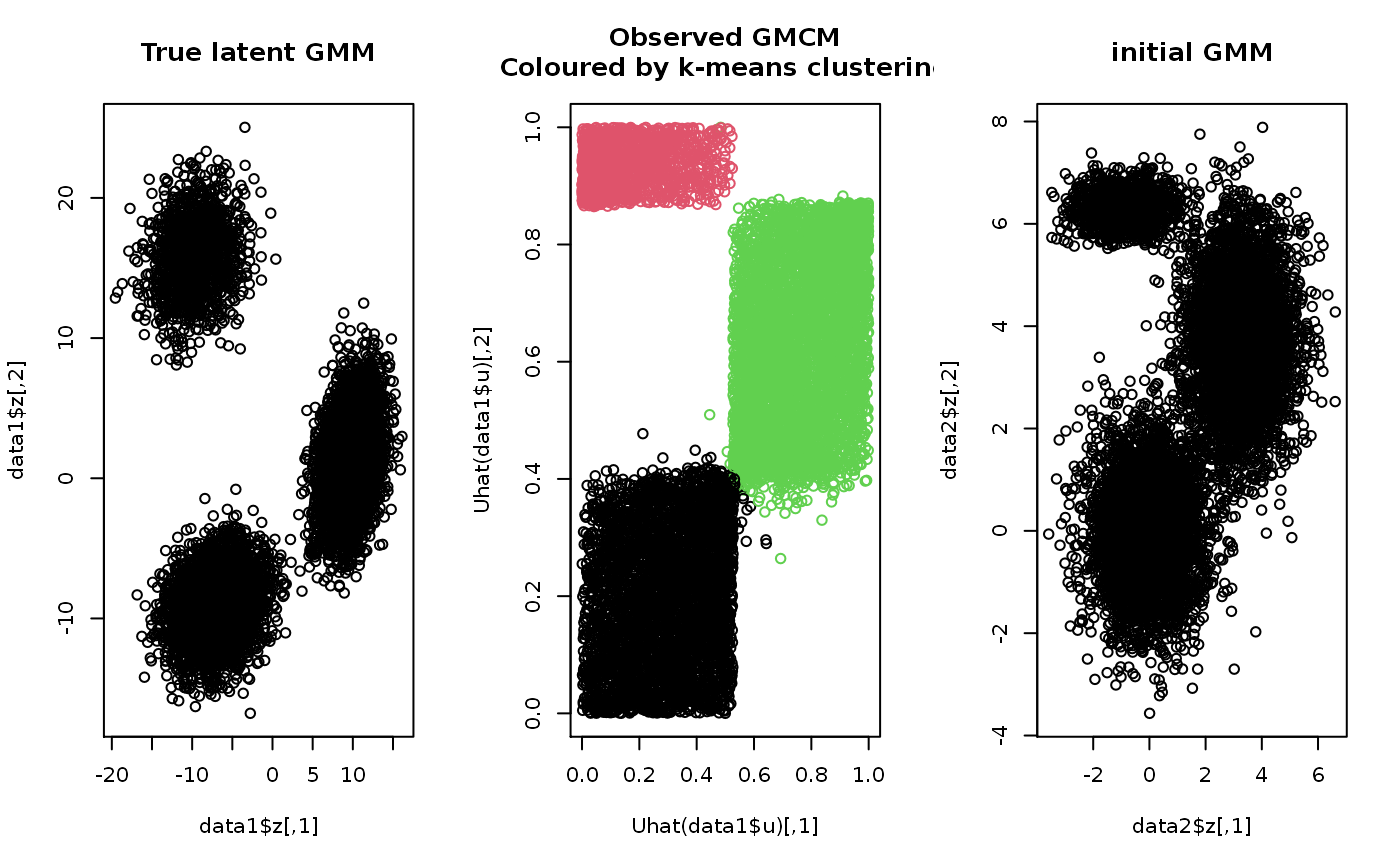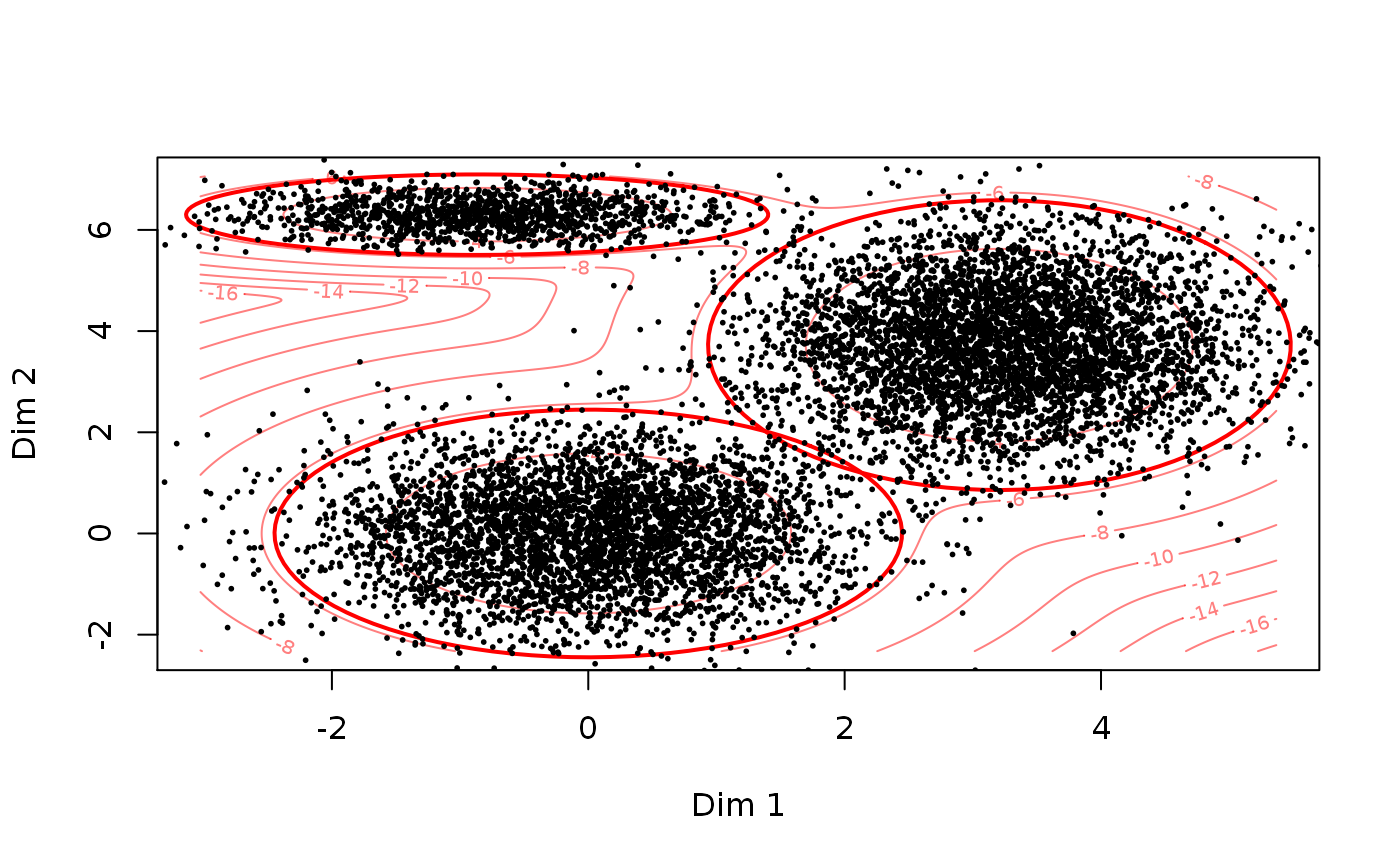This function uses a k-means algorithm to heuristically select suitable starting values for the general model.

choose.theta(u, m, no.scaling = FALSE, ...)

## Arguments

u A matrix of (estimates of) realizations from the GMCM. The number of components to be fitted. Logical. If TRUE, no scaling of the means and variance-covariance matrices is done. Arguments passed to kmeans.

## Value

A list of parameters for the GMCM model on the form described in rtheta.

## Details

The function selects the centers from the k-means algorithm as an initial estimate of the means. The proportional sizes of the clusters are selected as the initial values of the mixture proportions. The within cluster standard deviations are squared and used as the variance of the clusters within each dimension. The correlations between each dimension are taken to be zero.

## Note

The function uses the kmeans function from the stats-package.

## Author

Anders Ellern Bilgrau <anders.ellern.bilgrau@gmail.com>

## Examples

set.seed(2)

# Simulating data
data1 <- SimulateGMCMData(n = 10000, m = 3, d = 2)
obs.data <- Uhat(data1$u) # The ranked observed data # Using choose.theta to get starting estimates theta <- choose.theta(u = obs.data, m = 3) print(theta) #> theta object with d = 2 dimensions and m = 3 components: #> #>$pie
#>   pie1   pie2   pie3
#> 0.4002 0.1308 0.4690
#>
#> $mu #>$mu$comp1 #>  0 0 #> #>$mu$comp2 #>  -0.8668725 6.3009210 #> #>$mu$comp3 #>  3.207432 3.722536 #> #> #>$sigma
#> $sigma$comp1
#>      [,1] [,2]
#> [1,]    1    0
#> [2,]    0    1
#>
#> $sigma$comp2
#>           [,1]      [,2]
#> [1,] 0.8608994 0.0000000
#> [2,] 0.0000000 0.1056306
#>
#> $sigma$comp3
#>           [,1]     [,2]
#> [1,] 0.8622573 0.000000
#> [2,] 0.0000000 1.368835
#>
#>
# To illustrate theta, we can simulate from the model
data2 <- SimulateGMMData(n = 10000, theta = theta)

cols <- apply(get.prob(obs.data,theta),1,which.max)

# Plotting
par(mfrow = c(1,3))
plot(data1$z, main = "True latent GMM") plot(Uhat(data1$u), col = cols,
main = "Observed GMCM\nColoured by k-means clustering")
plot(data2$z, main = "initial GMM")# Alteratively, theta can simply be plotted to illustrate the GMM density par(mfrow = c(1,1)) plot(theta, add.ellipses = TRUE) points(data2$z, pch = 16, cex = 0.4)